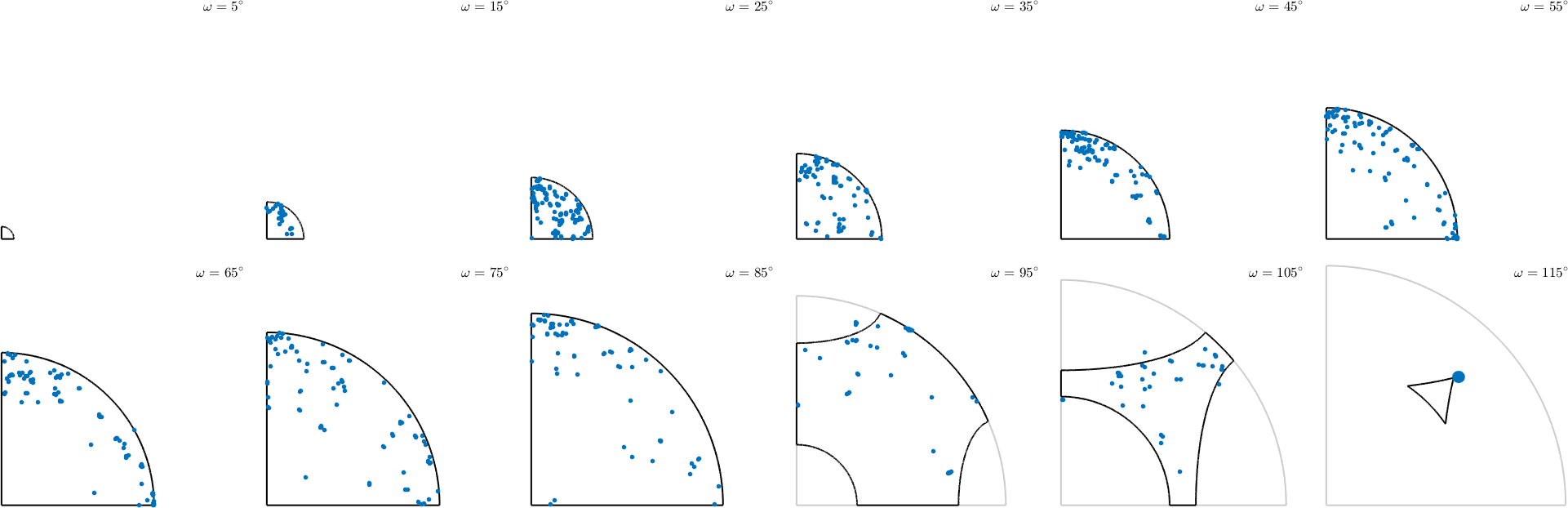Fundamental Regions edit page

Thanks to crystal symmetry the space of all rotations can be reduced to a so called fundamental or asymmetric region which has the property that each for orientation there is "exactly" one symmetrically equivalent orientation that is within the fundamental region. Those regions play an important role for visualizing orientations and ODFs as well as for the computation of axis and angle distributions of misorientations.

The space of all orientations can be imagined as a three dimensional ball with radius 180 degree. The distance of some point in the ball to the origin represent the rotational angle and the vector represents the rotational axis. In MTEX this can be represented as follows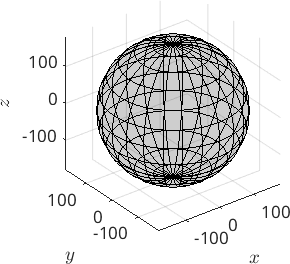Next we plot some orientations into this space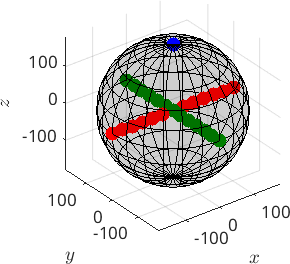An alternative view on the orientation space is by sections, e.g. sections along the Euler angles or along the rotational angle. The latter one should be demonstrated next: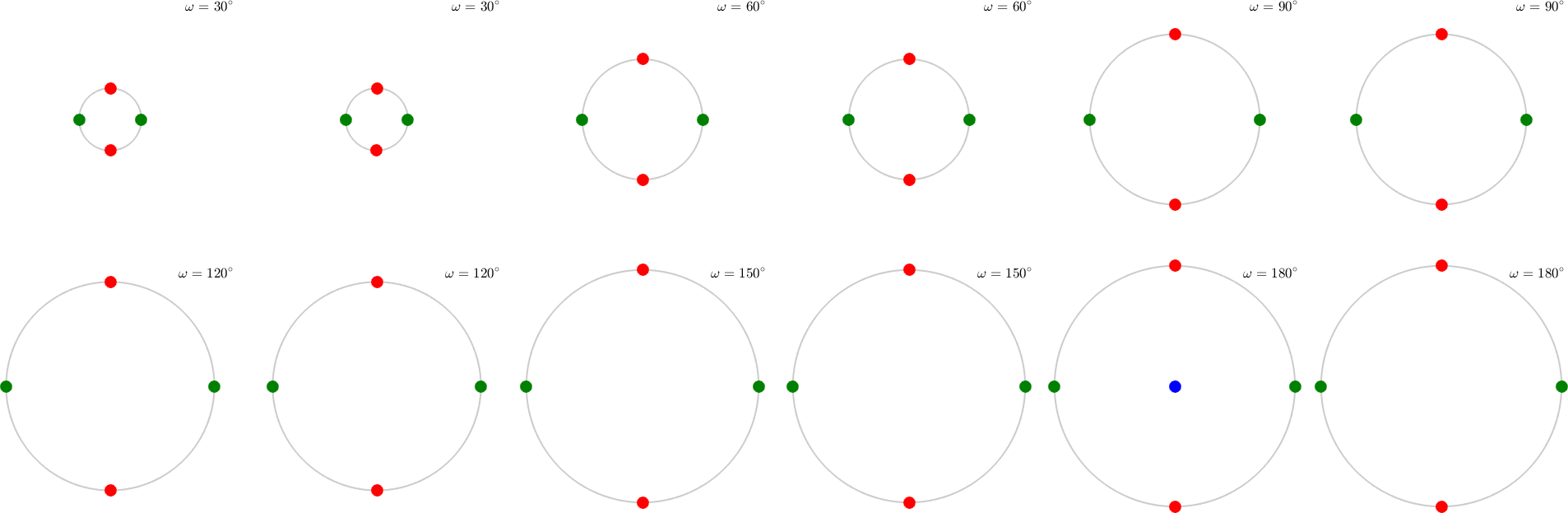## Crystal Symmetries

In case of crystal symmetries the orientation space can divided into as many equivalent segments as the symmetry group has elements. E.g. in the case of orthorombic symmetry the orientation space is subdivided into four equal parts, the central one looking like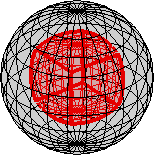As an example consider the following EBSD data set

we can visualize the Forsterite orientations by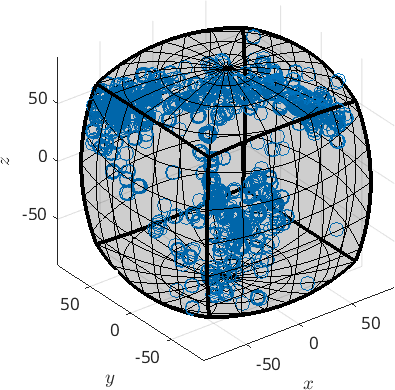We see that all orientations are automatically projected inside the fundamental region. In order to compute explicitly the represent inside the fundamental region we can do

## Change the center of the fundamental region

There is no necessity that the fundamental region has to be centered in the origin - it can be centered at any orientation, e.g. at the mean orientation of a grain.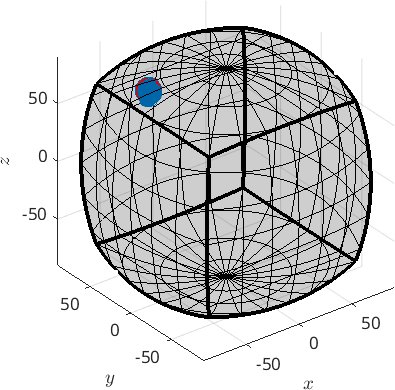## Fundamental regions of misorientations

Misorientations are characterised by two crystal symmetries. A corresponding fundamental region is defined by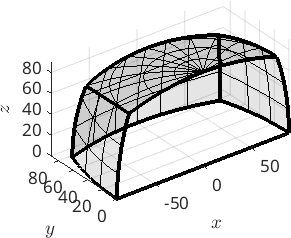Let plot grain boundary misorientations within this fundamental region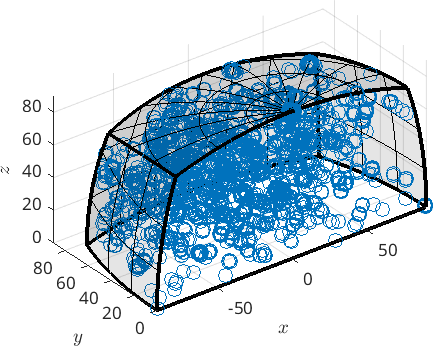## Fundamental regions of misorientations with antipodal symmetry

Note that for boundary misorientations between the same phase we can not distinguish between a misorientation and its inverse. This is not the case for misorientations between different phases or the misorientation between the mean orientation of a grain and all other orientations. The inverse of a misorientation is axis - angle representation is simply the one with the same angle but antipodal axis. Accordingly this additional symmetry is handled in MTEX by the keyword antipodal.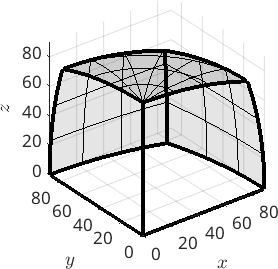We see that the fundamental region with antipodal symmetry has only half the size as without. In the case of misorientations between the same phase MTEX automatically sets the antipodal flag to the misorientations and plots them accordingly.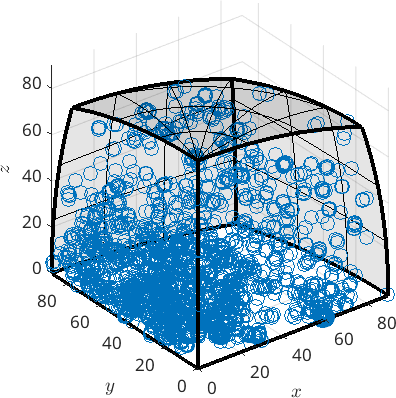If you want to avoid this you can remove the anitpodal flag by## Axis angle sections

Again we can plot constant angle sections through the fundamental region. This is done by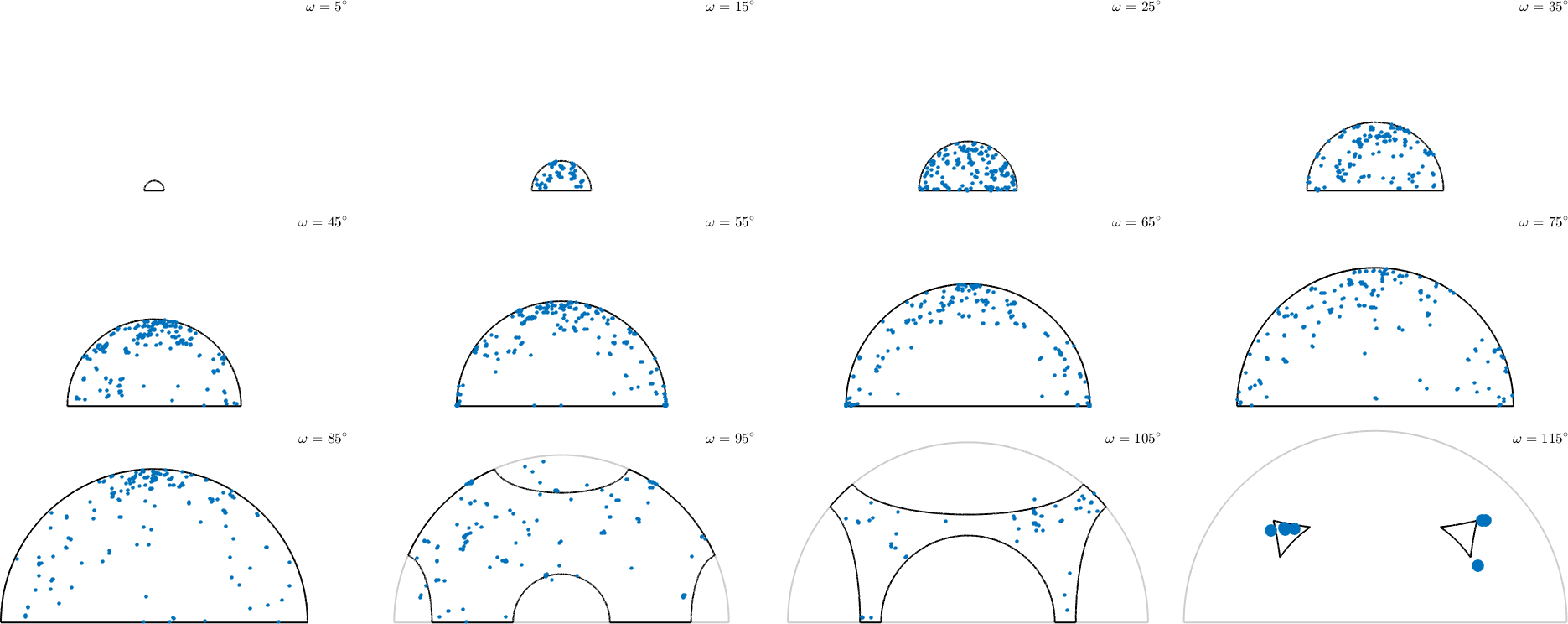Note that in the previous plot we distinguish between mori and inv(mori). Adding antipodal symmetry those are considered as equivalent